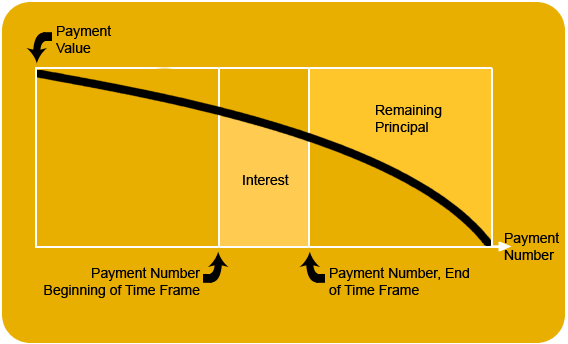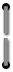Applications continue
##### Accumulated Interest Between Two Points, Remaining Principal
This calculation generates one of the more cumbersome sets of numbers found in actuarial amortizations (direct reduction loans) such as mortgages: the remaining principal to be paid, and the accumulated interest paid between any two points. Note that some mortgage holders round payments up to the nearest cent. Consequently the result of the remaining balance might differ from the actual amount as calculated on the HP-80.
The known values are: the payment numbers corresponding to the beginning and end of the time frame being analyzed, the total number of loan payments, interest rate per payment and amount of each payment (just the principal and interest exclusive of any insurance or tax amounts). The formula uses the constant storage location, therefore, any value stored there will be destroyed when the final key (the one that triggers the result) is pressed. Figure 3 illustrates a typical accumulated interest problem.Figure 3. Accumulated Interest Problem42